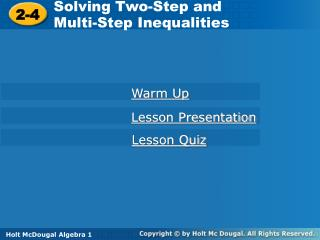DownloadDownload Presentation2-4

# 2-4

Télécharger la présentation## 2-4

- - - - - - - - - - - - - - - - - - - - - - - - - - - E N D - - - - - - - - - - - - - - - - - - - - - - - - - - -
##### Presentation Transcript

1. Solving Two-Step and Multi-Step Inequalities 2-4 Holt Algebra 1 Warm Up Lesson Presentation Lesson Quiz Holt McDougal Algebra 1

2. Warm Up Solve each equation. 1. 2x – 5 = –17 2. Solve each inequality and graph the solutions. 3. 5 < t + 9 4.

3. Objective Solve inequalities that contain more than one operation.

4. Steps to Solve Multistep Inequalities Use inverse operations to undo the operations in the inequality one at a time.

5. 45 + 2b > 61 –45 –45 b > 8 0 2 4 6 8 10 14 20 12 18 16 Example 1: Let’s do this together Solve the inequality and graph the solutions. 45 + 2b > 61 Since 45 is added to 2b, subtract 45 from both sides to undo the addition. 2b > 16 Since b is multiplied by 2, divide both sides by 2 to undo the multiplication.

6. Example 2: Let’s do this together Solve the inequality and graph the solutions. 8 – 3y ≥ 29

7. x + 5 < –6 –5 –5 –11 –20 –16 –12 –8 –4 0 Example 3: Lets do this together Solve the inequality and graph the solutions. Since x is divided by –2, multiply both sides by –2 to undo the division. Change > to <. Since 5 is added to x, subtract 5 from both sides to undo the addition. x < –11

8. Now you try Solve the inequality and graph the solutions. a.) –12 ≥ 3x + 6 b.)

9. To solve more complicated inequalities simplify the expressions on one or both sides by: • Using order of operations • Combining like terms • Using the Distributive Property

10. Example 2A: Simplifying Before Solving Inequalities Solve the inequality and graph the solutions. 2 – (–10) > –4t

11. –8 + 4x ≤ 8 +8 +8 10 –8 –10 –6 –4 0 2 4 6 8 –2 Example 2B: Simplifying Before Solving Inequalities Solve the inequality and graph the solutions. –4(2 – x) ≤ 8 –4(2 – x) ≤ 8 Distribute –4 on the left side. –4(2) – 4(–x) ≤ 8 Since –8 is added to 4x, add 8 to both sides. 4x ≤16 Since x is multiplied by 4, divide both sides by 4 to undo the multiplication. x ≤ 4

12. Example 2C: Simplifying Before Solving Inequalities Solve the inequality and graph the solutions.

13. Check It Out! Example 2a Solve the inequality and graph the solutions. d.) 3 + 2(x + 4) > 3 c.) 2m+ 5 > 52 e.)

14. daily cost at We Got Wheels Cost at Rent-A-Ride must be less than \$0.20 per mile # of miles. plus times 55 < 38 m + 0.20  Example 3: Application To rent a certain vehicle, Rent-A-Ride charges \$55.00 per day with unlimited miles. The cost of renting a similar vehicle at We Got Wheels is \$38.00 per day plus \$0.20 per mile. For what number of miles is the cost at Rent-A-Ride less than the cost at We Got Wheels? Let m represent the number of miles. The cost for Rent-A-Ride should be less than that of We Got Wheels. Rent-A-Ride costs less when the number of miles is more than 85. 85 < m

15. Check It Out! Example 3 The average of Jim’s two test scores must be at least 90 to make an A in the class. Jim got a 95 on his first test. What grades can Jim get on his second test to make an A in the class?

16. HOMEWORK PG. 123-125 #16-48(evens), 37, 59, 63-66